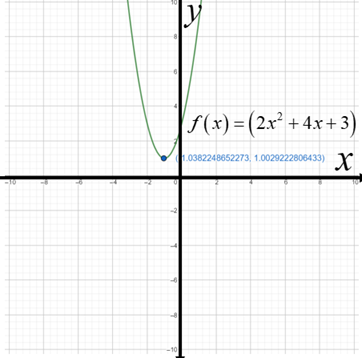# To Express: The quadratic function in standard form.### Precalculus: Mathematics for Calcu...

6th Edition
Stewart + 5 others
Publisher: Cengage Learning
ISBN: 9780840068071### Precalculus: Mathematics for Calcu...

6th Edition
Stewart + 5 others
Publisher: Cengage Learning
ISBN: 9780840068071

#### Solutions

Chapter 3.1, Problem 17E

a.

To determine

## To Express: The quadratic function in standard form.

Expert Solution

the quadratic function is expressed in standard form as  f(x)=2(x+1)2+1

### Explanation of Solution

Given: The function is f(x)=(2x2+4x+3)

Calculation:

The quadratic function f(x)=(2x2+4x+3) is expressed in standard form as:

f(x)=a(xh)2+k , by completing the square. The graph of the function f is a parabola with vertex (h,k)

The parabola opens upwards if a>0 .

Solve the function:

f(x)=(2x2+4x+3)f(x)=2(x2+2x)+3                         [factor 2 from the x terms] f(x)=2(x2+2x+1)+3(21)        [complete the square : add 1 inside parentheses, subtract (21) outside] f(x)=2(x+1)2+1                             [factor and multiply]

On comparing the above equation with standard form f(x)=a(xh)2+k ,

Therefore, the quadratic function is expressed in standard form as  f(x)=2(x+1)2+1

b.

To determine

### To Find: The vertex, x−intercept and y−intercept

Expert Solution

the vertex is (h,k)=(1,1) .

there is no x-intercept .

y-intercept=f(0)=3

### Explanation of Solution

Given: The function is f(x)=(2x2+4x+3)

Calculation:

The quadratic function f(x)=(2x2+4x+3) is expressed in standard form as:

f(x)=2(x+1)2+1

by completing the square. The graph of the function f is a parabola with vertex (h,k) , the vertex is (h,k)=(1,1) .

The y-intercept is given when x=0 , so y-intercept=f(0)=3

From the standard form it is observed that the graph is a parabola that opens upward and has vertex (h,k)=(1,1) . As an aid to sketching the graph, find the intercepts.

The y-intercept=f(0)=3 . To find the x-intercepts , set f(x)=0 and factor the resulting equation, it is observed that there is no x-intercept .

c.

To determine

Expert Solution

### Explanation of Solution

Given: The function is f(x)=(2x2+4x+3)

Graph:

The standard form of the function is:

f(x)=2(x+1)2+1

From the standard form it is observed that the graph is a parabola that opens downward and has vertex (1,1) . As an aid to sketching the graph, find the intercepts. The y-intercept=f(0)=3 and there is no x-intercept . The graph f is sketched in the figure below.

Use graphing calculator to graph the function: f(x)=(2x2+4x+3)### Have a homework question?

Subscribe to bartleby learn! Ask subject matter experts 30 homework questions each month. Plus, you’ll have access to millions of step-by-step textbook answers!